Test: Radiative Heat Transfer Level - 2

# Test: Radiative Heat Transfer Level - 2

Test Description

## 20 Questions MCQ Test Heat Transfer | Test: Radiative Heat Transfer Level - 2

Test: Radiative Heat Transfer Level - 2 for Chemical Engineering 2022 is part of Heat Transfer preparation. The Test: Radiative Heat Transfer Level - 2 questions and answers have been prepared according to the Chemical Engineering exam syllabus.The Test: Radiative Heat Transfer Level - 2 MCQs are made for Chemical Engineering 2022 Exam. Find important definitions, questions, notes, meanings, examples, exercises, MCQs and online tests for Test: Radiative Heat Transfer Level - 2 below.
Solutions of Test: Radiative Heat Transfer Level - 2 questions in English are available as part of our Heat Transfer for Chemical Engineering & Test: Radiative Heat Transfer Level - 2 solutions in Hindi for Heat Transfer course. Download more important topics, notes, lectures and mock test series for Chemical Engineering Exam by signing up for free. Attempt Test: Radiative Heat Transfer Level - 2 | 20 questions in 45 minutes | Mock test for Chemical Engineering preparation | Free important questions MCQ to study Heat Transfer for Chemical Engineering Exam | Download free PDF with solutions
 1 Crore+ students have signed up on EduRev. Have you?
Test: Radiative Heat Transfer Level - 2 - Question 1

### What is net radiation heat exchange per square meter per unit time for two very large plates at temperature values of 800 K and 500 K? Emissivities of hot and cold plates are 0.8 and 0.6 respectively. Stefan-Boltzmann constant is - 5.67x10-8W/m2K4

Detailed Solution for Test: Radiative Heat Transfer Level - 2 - Question 1

For two very large parallel plates, the net radiation heat exchange (using thermal circuit) is given by,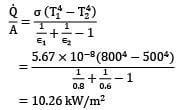= 10.26 kW/m2

Test: Radiative Heat Transfer Level - 2 - Question 2

### The spectral hemispherical absorptivity of an opaque surface is as shown.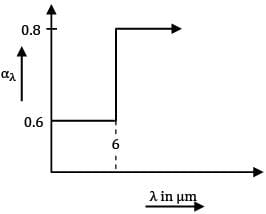The surface is exposed to ir-radiation for which the spectral distribution is as shown.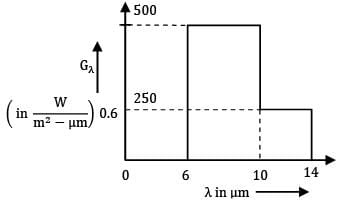What is the total hemispherical absorptivity of surface?

Detailed Solution for Test: Radiative Heat Transfer Level - 2 - Question 2

Total absorptivity is defined as

α = Ga/G

Where Ga = ∫oooαλGλdλ

Ga = ∫06 0.6 x 0 dλ + ∫610 0.8 x 500 dλ + ∫1014 0.8 x 250 dλ + ∫1400 0.8 x 0 dλ

∴ Ga = 0 + 0.8 x 500 x (10-6) + 0.8 x 250 x (14-10) + 0

Ga = 2400 W/m2 ...①

and

G = ∫000 Gλdλ

I.e. G = Area under graph Gλ Vs λ

G = 500 x (10-6) + 250 x (14-10)

G = 2000 + 1000

G = 3000 W/m2 ...②

Therefore, from ① & ②

α = Ga/G = 2400/3000

α = 0.8

Test: Radiative Heat Transfer Level - 2 - Question 3

### Two grey surfaces that form an enclosure exchange heat with one another by thermal radiation. Surface 1 has a temperature of 400 K, an area of and a total emissivity of 0.4. Surface 2 has a temperature of 600 K, area of and a total emissivity of 0.6. If the view factor is 0.3, the rate of radiation heat transfer between the two surfaces is

Detailed Solution for Test: Radiative Heat Transfer Level - 2 - Question 3

Using thermal circuit, the net heat exchange between two surfaces is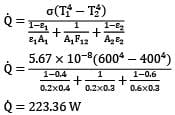Q = 223.36 W

Test: Radiative Heat Transfer Level - 2 - Question 4

Which of the following statements is false regarding spectral distribution of thermal radiation emitted by a grey surface P. Monochromatic emissivity is independent of wavelength Q. Total emissivity is equal to monochromatic emissivity.

R. (monochromatic emissive power) Vs λ( graph for the surface at a

given temperature is scaled down version of the graph for black body at same temperature.

S. The wavelength corresponding to maximum monochromatic emissive power is same as that of grey surface at same temperature.

Detailed Solution for Test: Radiative Heat Transfer Level - 2 - Question 4

A grey surface is one for which monochromatic emissivity ελ is independent of wavelength (λ ) But total emissivity (ε) of a surface is given as

ε = E/Eb

Where E & Eb represent total emissive power of the surface and a black surface at same temperature.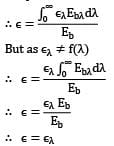But as ελ ≠ f(λ)∴ ε = ελEb/Eb

∴ ε = ελ

i.e. total emissivity is equal to monochromatic emissivity. Hence statement Q is also correct.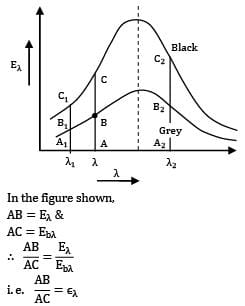In the figure shown,

AB = Eλ &

AC = E

∴ AB\Ac = Eλ/E

i.e, AB\AC = ελ

i.e. ratio of line segment AB and AC is monochromatic emissivity for surface corresponding to wavelength (λ) But as (ελ≠f(λ))

this ratio is same for all λ

i.e, A1B1/A1C1 = AB/AC = A2B2/A2C2

Thus graph of grey surface is simply scaled down version of graph of black surface. This also suggests that corresponding to maxima point for both surfaces is same.

Hence statement R & S are also correct.

Thus all statements are correct & option (D) is the correct answer.

Test: Radiative Heat Transfer Level - 2 - Question 5

Two infinitely large parallel plates 1 & 2, facing each other are separated by a very small gap. The plates are exchanging heat by thermal radiation and gap between them is filled with non-participating medium. Surface 1 is a black surface with temperature equal to and surface 2 is a grey surface with temperature equal to and emissivity equal to 0.8. Find out the radiosity for surface 2 in kW/m2

Detailed Solution for Test: Radiative Heat Transfer Level - 2 - Question 5

Consider radiation heat exchange between the surface as shown.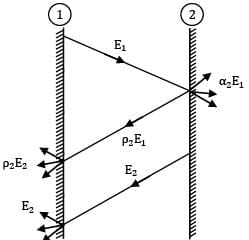Black Grey,ε2 = 0.8

T1 = 800 K T2 = 500 K

Therefore radiosity for surface 2 is given as

J2 = E2 + ρ2E1

J2 = E2 + (1-α2)E1

By Kirchoff’s law, α2 = ε2

∴ J2 = (1-ε2)E1+ E2

As ① is a black surface,

E1 = σT14

and ② is a gray surface,

E2 = ε2σT24

∴ J2 = (1-ε2)σT14 + ε2σT24

J2 = σ[(1-ε)T14 + ε2T24]

J2 = 5.67 x 10-8 + [(1-0.8) x 8004 + 0.6 x 5004]

J2 = 6.77 kW/m2

Test: Radiative Heat Transfer Level - 2 - Question 6

Two large parallel grey plates with a small gap between them, exchange radiation at the rate of when their emissivities are 0.5 each. By coating one plate, its emissivity is reduced to 0.25. Temperatures remain unchanged. The new rate of heat exchange shall become

Detailed Solution for Test: Radiative Heat Transfer Level - 2 - Question 6

By thermal circuits, net radiation heat exchange between two surfaces can be written as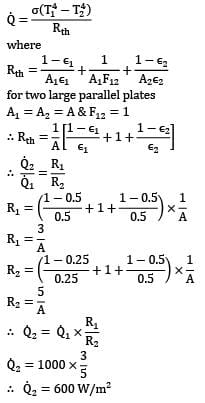WhereFor two large parallel plates

A1 = A2 = A & A12 = 1

∴ Q2/Q1 = R1/R2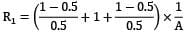R1 = 3/A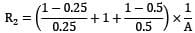R2 = 5/A

∴ Q2 =Q1 x R1/R2

Q2 = 1000 x ⅗

∴ Q2 = 600 W/m2

Test: Radiative Heat Transfer Level - 2 - Question 7

An enclosure consists of four surfaces 1, 2, 3 and 4. The view factors for radiation heat transfer (where the subscripts 1, 2, 3, 4 refer to the respective surfaces) are F11 = 04 and F13 = 0.25. The surface areas A1 and A4 are 4m2 respectively. The view factor is

Detailed Solution for Test: Radiative Heat Transfer Level - 2 - Question 7

F11 + F12 + F13 + !4 = 1

0.1 + 0.4 + 0.25 + F14 = 1

∴ F14 = 0.25

From reciprocity theorem, A1F14 = A4F41

4 x 0.25 = 2 x F41

∴ F41 = 0.5

Test: Radiative Heat Transfer Level - 2 - Question 8

The net radiation heat exchange rate between a heating element at a temperature of 800oC and inner wall of a furnace maintained at . The heat exchange rate when the element temperature is increased to 1000oC for the same furnace temperature is

Detailed Solution for Test: Radiative Heat Transfer Level - 2 - Question 8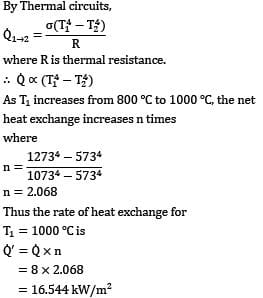By Thermal circuits,

Where R is thermal resistance.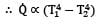As T2 increases from 800oC to 1000oC, the net heat exchange increases n times

Where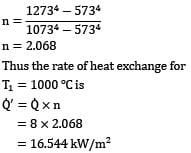n = 2.068

Thus the rate of heat exchange for

T1 = 10000C

Q’ = Q x n

= 8 x 2.068

= 16.544 kW/m2

Test: Radiative Heat Transfer Level - 2 - Question 9

A filament of 75 W light bulb may be considered as a black body radiating into a black enclosure at . The filament diameter is 0.1 mm and length is 5 cm. Considering only radiation, the filament temperature is

Detailed Solution for Test: Radiative Heat Transfer Level - 2 - Question 9

At steady state electric power supply to the bulb is equal to net radiation heat exchange between bulb and enclosure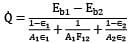here ∈1 = ∈2 = 1 and

F12 = 1; filament is completely enclosed

Q = A1F12σ(T14 - T24)

Q = (πDL) x 1 x 5.67 x 10-8 (T14 - 3434)

= 75 = 3.14 x 0.0001 x 0.05 x 1 x 5.67 x 10-8 (T14= 3434)

T1 = 3029.4 K

Test: Radiative Heat Transfer Level - 2 - Question 10

Consider a surface at 0oC that may be assumed to be a blackbody in an environment at . If of radiation is incident on the surface, the radiosity of this black surface is

Detailed Solution for Test: Radiative Heat Transfer Level - 2 - Question 10

By definition radiosity is the rate at which a thermal radiation levels a surface per unit area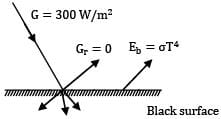Ga = 300 W/m2

As surface is black, it completely absorbs the radiation incident on it,

Therefore

Gr = 0

By Stefan Boltzmann Law

Eb = σT4

= 5.67 x 10-8 x 2734

Eb = 314.94 W/m2

J = Eb +Gr

J = 314.94 W/m2

Note that Eb>G because the difference Eb - Ga must be equal to rate of heat convection from the environment to surface.

Test: Radiative Heat Transfer Level - 2 - Question 11

A solar flux of intensity directly strikes a space vehicle surface which has an absorptivity of 0.4 and emissivity of 0.6. The equilibrium temperature of this surface in space at 0 K is

Detailed Solution for Test: Radiative Heat Transfer Level - 2 - Question 11

For equilibrium,

Rate of heat absorbed = Rate of heat emitted

As the space vehicle is radiating to space at 0 K

∴ αAGr = ∊ A σ T14

⇒ 0.4 x 1400 = 0.6 x 5.67 x 10-8 x T14

⇒ T1 = 358.2 K

Test: Radiative Heat Transfer Level - 2 - Question 12

The shape factor of a cylindrical cavity (open from top) with respect to itself is __________. L & D are length and diameter of the cavity respectively.

Detailed Solution for Test: Radiative Heat Transfer Level - 2 - Question 12

The cavity is the shape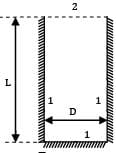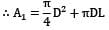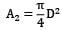2 is a planar surface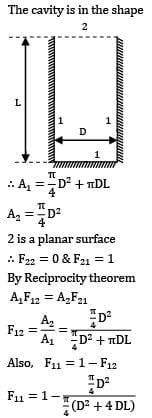∴ F22 = 0 & F21 = 1

By Reciprocity theorem

A1A12 = A2F21

Also, F11 = 1- F12F11 = 1 - D2/D2+4DL

1-D/D+4L

∴ F11 = 4L/D+4L

Test: Radiative Heat Transfer Level - 2 - Question 13

What is the view factor for inclined parallel plates of equal width and a common edge? The width of plates to the plane of paper is b. (b >>L)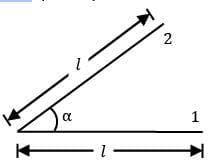Detailed Solution for Test: Radiative Heat Transfer Level - 2 - Question 13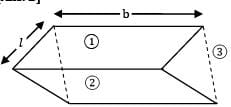Let us imagine the rectangular gap as a surface ③. !s the energy going out of triangular gaps can be neglected, but for rectangular gap, it is quite significant

Length of side 3 = 2 ℓ sin(α/2)

∴ A3 = (2 ℓ sin α/2 x b) = 2 ℓb sin (α/2)

A1 = ℓ x b = ℓb

A2 = ℓ x b = ℓlb

As 3 is a planar surface

F33 = 0

Also F31 + F32 + F33 =1

But because of symmetric arrangement of ①

& ② with respect to ③

F31 = F32

∴ F31 = F32 = 0.5

By Reciprocity theorem

A1A13 = A3A31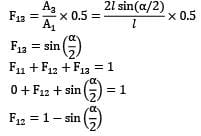F13 = ain(α/2)

F11 + F12 + F13 = 1

0 + F12 + sin (α/2) = 1

F12 = 1-sin(α/2)

Test: Radiative Heat Transfer Level - 2 - Question 14

Two very large parallel plates separated by vacuum are maintained at uniform temperatures T1= 750K & T2=500K and have emissivities ε1= 0.85 and ε2 = 0.7 respectively. If a thin aluminium sheet with same emissivity on both sides is placed in between the plates, the rate of heat transfer between the plates reduces by 90%. The emissivity of aluminium sheet is given as

Detailed Solution for Test: Radiative Heat Transfer Level - 2 - Question 14

By thermal circuits,

Q ∝ 1/R

Where R is resistance to radiation heat exchange between the surfaces.

When there is no sheet between the plates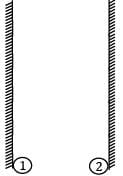T1 = 750 K T2 = 500 K

ε1 = 0.85 ε20.7

R1 = 1-ε1/Aε1+1/A+1-ε2/Aε2

R1 = 1-0.85/0.854A+1/A+1-0.7/0.7A

R1 = 1.605/A

When a sheet is placed between the surfaces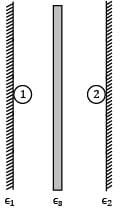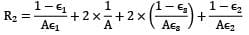Where εs is the emissivity of single shield

surface

∴ R2 = 2.605/A+2(1-εs)/Aεs

∴ Q2/Q1 = R1/R2

It is given that by introducing the sheet in between the plates reduces the heat exchange by 90%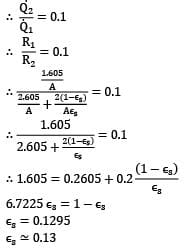∴ Q2/Q1 = 0.1

∴ R1/R2 = 0.1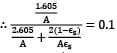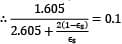∴ 1.605 = 0.2605 + 0.2 (1-εs)/εs

6.7225 εs = 1-εs

εs = 0.1295

εs ≃ 0.13

Test: Radiative Heat Transfer Level - 2 - Question 15

For the radiation between two infinite parallel planes of emissivity respectively, which one of the following is the expression for emissivity factor or equivalent emissivity?

Detailed Solution for Test: Radiative Heat Transfer Level - 2 - Question 15

The equivalent thermal resistance between two gray bodies is given by

Rth = 1-ε1/A1ε1+1/A1F12+1-ε2/A2ε2

Now for infinite parallel panes, F12 =1

A1 = A2 = A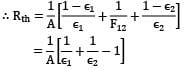For unit area, A = 1 m2

Rth = 1/ε1+1/ε2-1

Hence equivalent emissivity

Εe = 1/Rth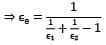Test: Radiative Heat Transfer Level - 2 - Question 16

Determine the view factors from the base of the pyramid shown in figure to each of its four side surfaces. The base of the pyramid is a square and its side surfaces are isosceles triangles.

Detailed Solution for Test: Radiative Heat Transfer Level - 2 - Question 16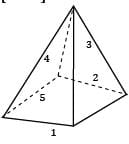The base of the pyramid (side 1) and the other four surfaces form an enclosure From symmetry, we have.

F12 = F13 = F14 = F15

Again we have,

F11+F12+F13+F14+F15 = 1

Now F11 = 0

∴ F12 = F13 = F14 = F15 = 0.25

Test: Radiative Heat Transfer Level - 2 - Question 17

Determine for the following configuration. Long inclined plates (point B is directly above the center of )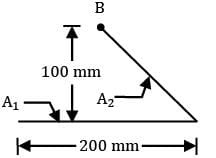Detailed Solution for Test: Radiative Heat Transfer Level - 2 - Question 17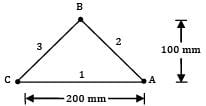Closing the remaining side by a hypothetical surface 3, we have

F11 + F12 + F13 = 1

F11 = 0; By symmetry we have,

F12 = F13

∴ F12 = F13 = 0.5

A1F12 = A2F21(by reciprocity relation)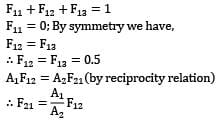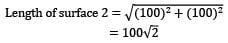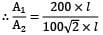(considering depth of plates ⊥ to plane of paper to be l)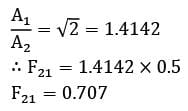∴ F21 = 1.4142 x 0.5

F21 = 0.707

Test: Radiative Heat Transfer Level - 2 - Question 18

Consider surfaces ① & ② as shown in the figure.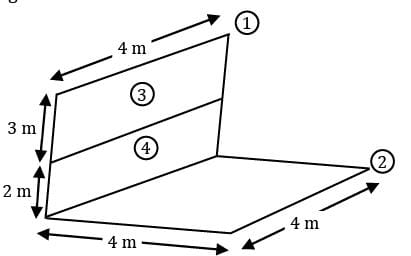Surface ① is divided into two parts ③ & ④. Which of the following options is correct expression for F12

Detailed Solution for Test: Radiative Heat Transfer Level - 2 - Question 18

Q1➝2 = Q3➝2 + Q4➝2

Q1➝2 /Q1 = Q3➝2 /Q1+ Q4➝2/Q1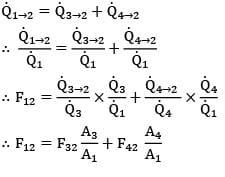Test: Radiative Heat Transfer Level - 2 - Question 19

What is the equivalent emissivity for radiant heat exchange between a small body ( in a very large enclosure (emissivity = 0.5)?

Detailed Solution for Test: Radiative Heat Transfer Level - 2 - Question 19

If one surface is completely enclosed by another surface,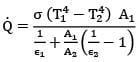If surface 2 is very large,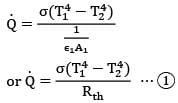Also we have q = εeσ(T14 - T24) ...②

From ① & ② εe = 1/Rth

But Rth = 1 /ε1A1

∴ εe = ε1 = 0.4

Test: Radiative Heat Transfer Level - 2 - Question 20

Determine the percentage of radiation emitted by the sun within the visible spectrum ( Wavelengths from 0.4 μm (violet) to 0.76 μm(red), considering the sun to be black body and its effective surface temperature to be 5800 K. Given black body radiation function for 2320μmk is 0.124509 and for 4408μmk is 0.550015 .

Detailed Solution for Test: Radiative Heat Transfer Level - 2 - Question 20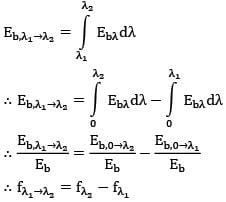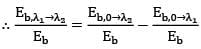∴ fλ1➝λ2 = fλ2 - fλ1

Where fλ is black body radiation function for wavelength λ.

∴ f0.4-0.76 = f0.76 - f0.4

= 0.550015 - 0.124509

= 0.4255

∴ i.e, ≃ 42.58%

## Heat Transfer

58 videos|70 docs|85 tests
 Use Code STAYHOME200 and get INR 200 additional OFF Use Coupon Code